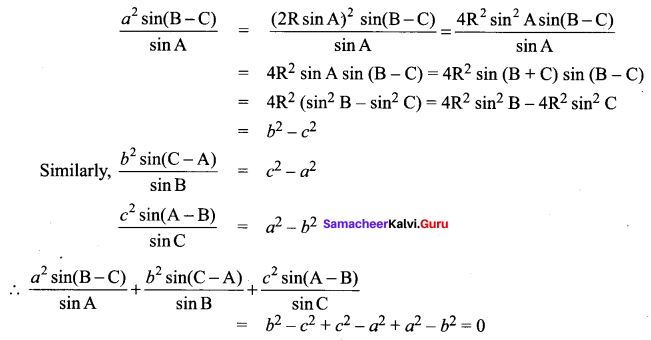## Tamilnadu Samacheer Kalvi 11th Maths Solutions Chapter 3 Trigonometry Ex 3.9

Question 1.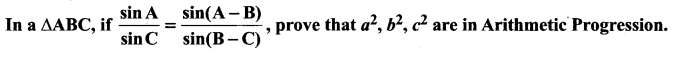Solution: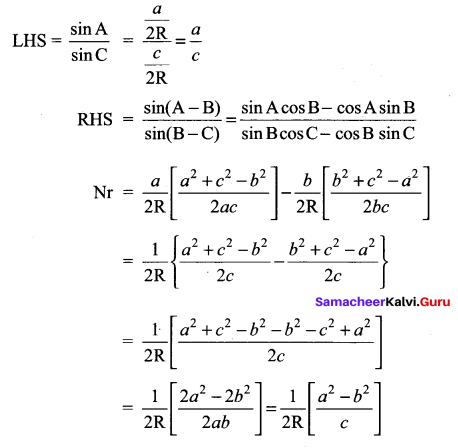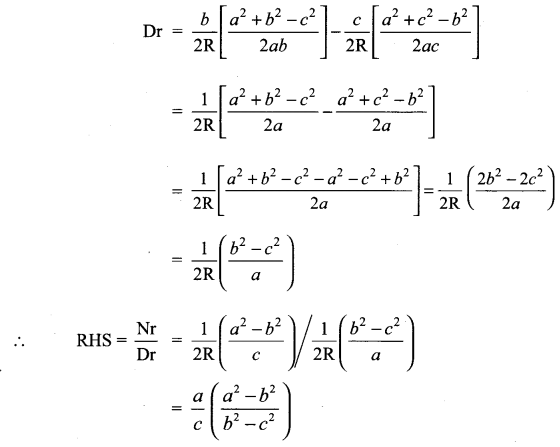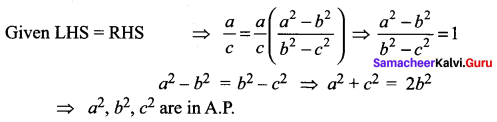Question 2.
The angles of a triangle ABC, are in Arithmetic Progression and if b : c = $$\sqrt{3}: \sqrt{2}$$, find ∠A.
Solution: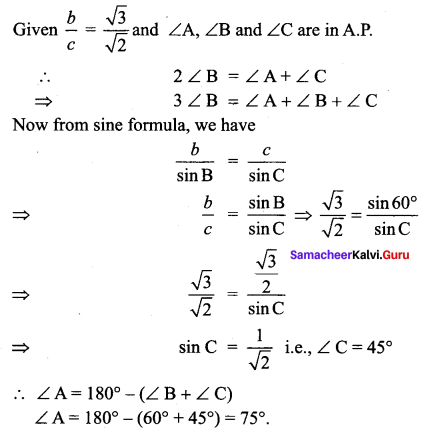Question 3.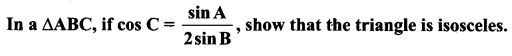Solution: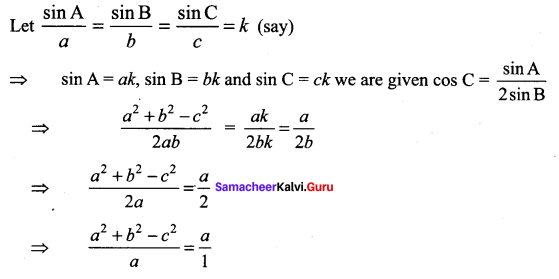⇒ a2 + b2 – c2 = a2 ⇒ b2 – c2 = a2 – a2
⇒ b2 – c2 = 0 ⇒ b = c
∴ ∆ ABC is isosceles

Question 4.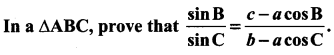Solution: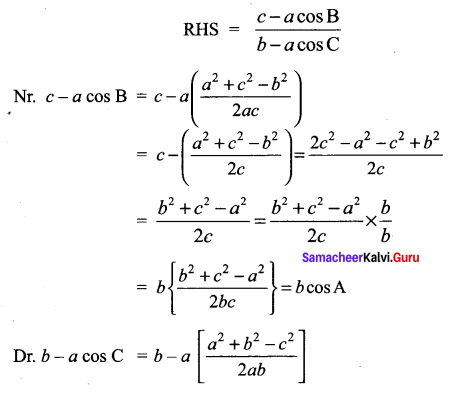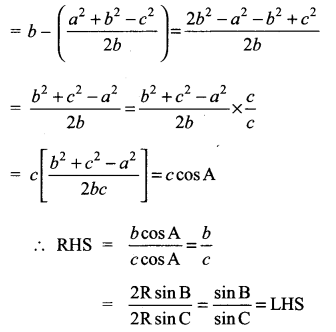Question 5.
In a ∆ABC, prove that a cos A + b cos B + c cos C = 2a sin B sin C.
Solution:
LHS = a cos A+ 6 cos B + c cos C
Using sine formula, we get k sin A cos A + k sin B cos B + k sin C cos C k
= $$\frac{k}{2}$$ [2 sin A cos A + 2 sin B cos B + 2 sin C cos C]
= $$\frac{k}{2}$$ [sin 2A + sin 2B + sin 2C]
= $$\frac{k}{2}$$ [2 sin (A + B) . cos (A – B) + 2 sin C . cos C]
= $$\frac{k}{2}$$ [2 sin (A – B) . cos (A – B) + 2 sin C . cos C]
= $$\frac{k}{2}$$ [2 sin C . cos (A – B) + 2 sin C . cos C]
= k sin C [cos(A – B) + cos C]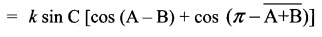= k sin C [cos (A – B) – cos (A + B)]
= k sin C . 2 sin A sin B
= 2k sin A . sin B sin C
= 2a sin B sin C = RHS

Question 6.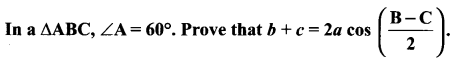Solution: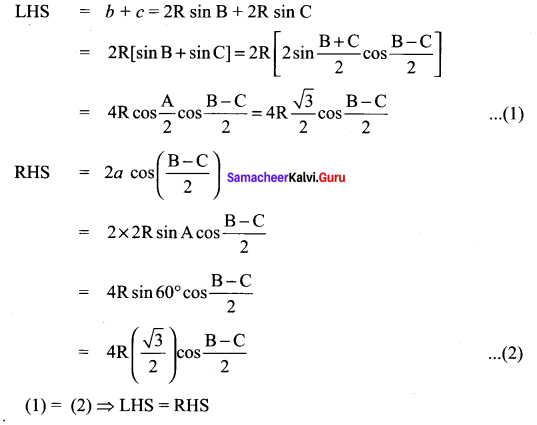Question 7.
In a ∆ ABC, prove the following.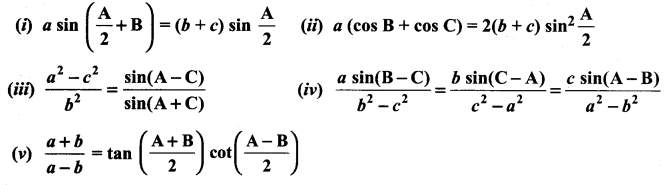Solution: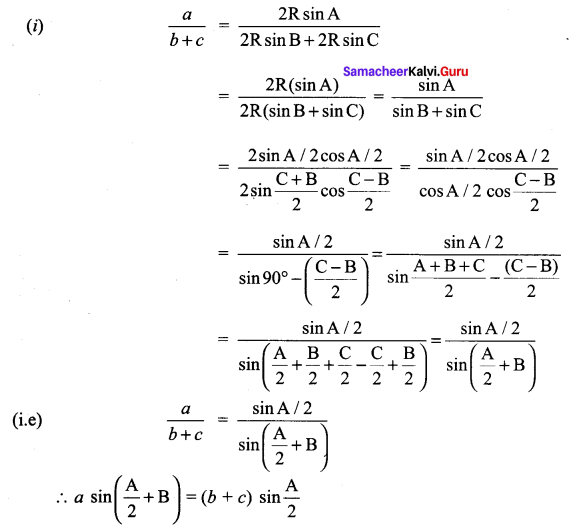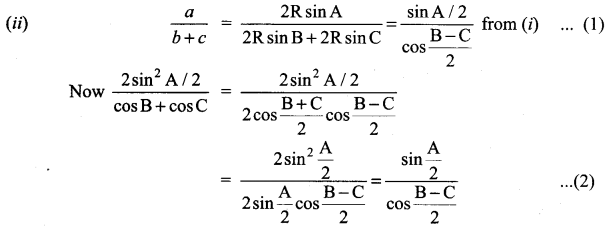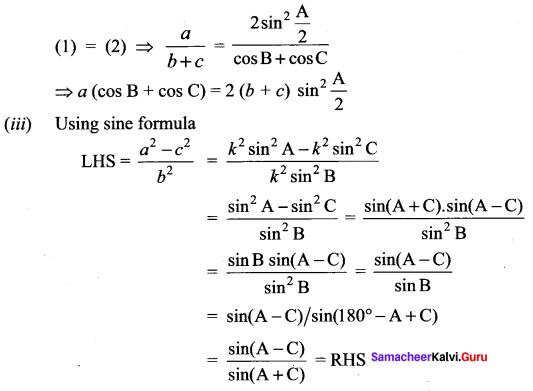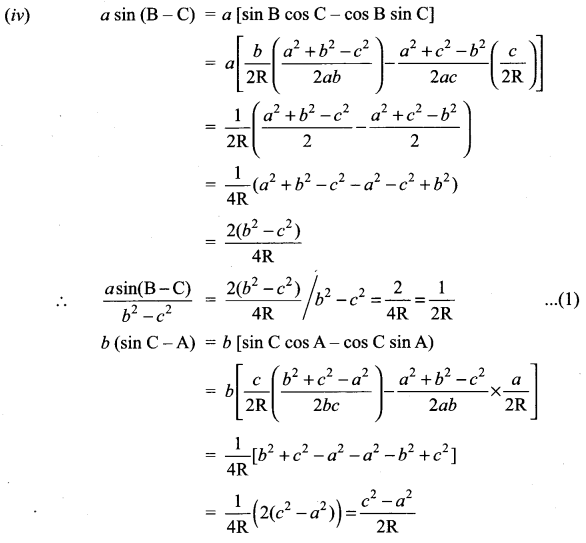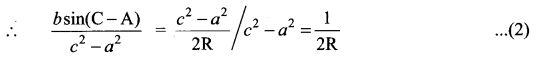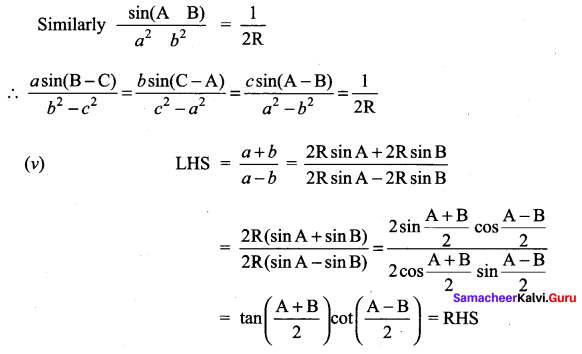Question 8.
In a ∆ABC, prove that (a2 – b2 + c2) tan B = (a2 + b2 – c2)tan C
Solution: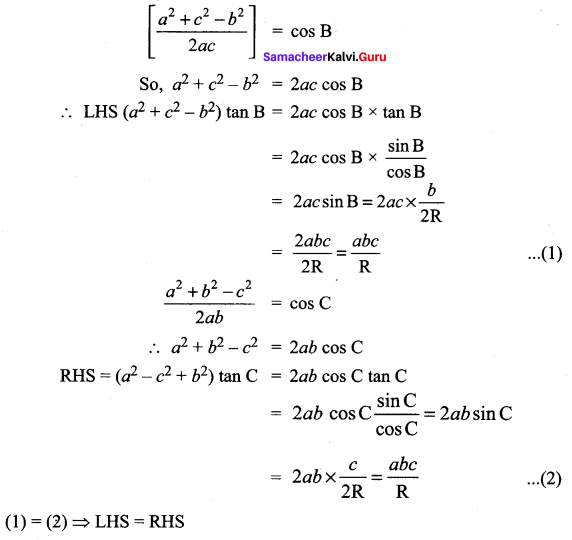Question 9.
An Engineer has to develop a triangular shaped park with a perimeter 120 m in a village. The park to be developed must be of maximum area. Find out the dimensions of the park.
Solution:
Given, the perimeter of triangular shaped park = 120 m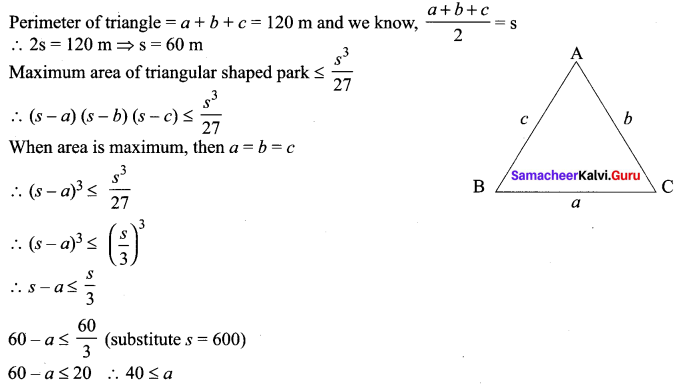All sides of a triangular part would be 40 m.
i.e., a = 40 m,
b = 40 m,
c = 40 m.Question 10.
A rope of length 12 m is given. Find the largest area of the triangle formed by this rope and find the dimensions of the triangle so formed.
Solution:
The largest triangle will be an equilateral triangle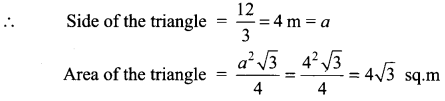Question 11.
Derive Projection formula from
(i) Law of sines,
(ii) Law of cosines.
Solution:
(i) To Prove a = b cos c + c cos B
Using sine formula
RHS = b cos C + c cos B
= 2R sin B cos C + 2R sin C cos B
= 2R [sin B cos C + cos B sin C]
= 2R sin (B + C) = 2R [sin π – A)
= 2R sin A = a = LHS

(ii) To prove a = b cos c + c cos B
Using cosine formula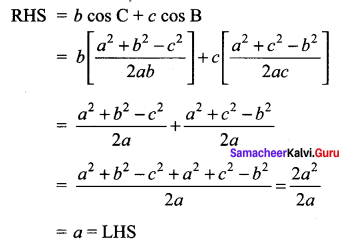### Samacheer Kalvi 11th Maths Solutions Chapter 3 Trigonometry Ex 3.9 Additional Questions

Question 1.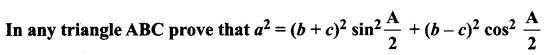Solution: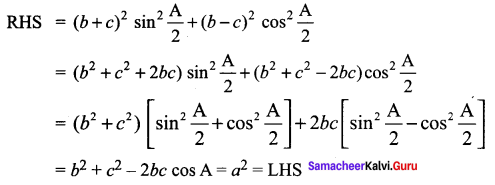Question 2.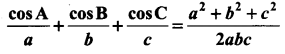Solution: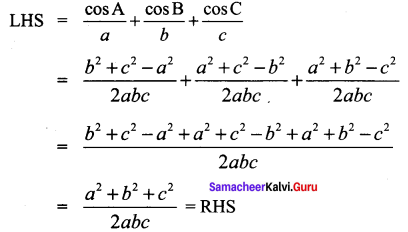Question 3.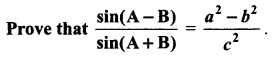Solution: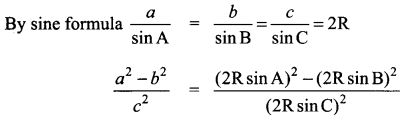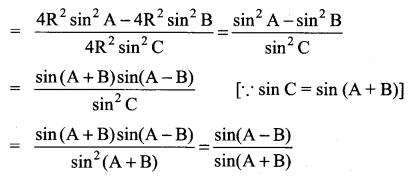Question 4.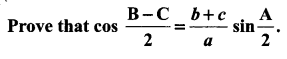Solution:Question 5.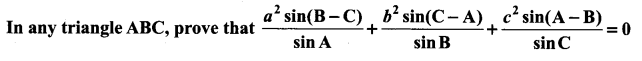Solution: